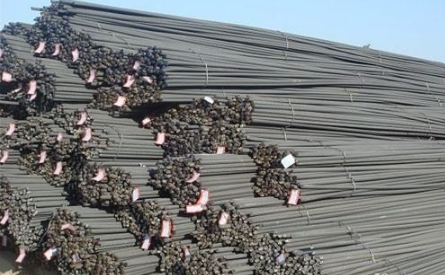2017-3-7 15:28 中国泵阀制造网 点击：11527

1. 钢材的范围定尺 是节省材料的一种有效措施。范围定尺就是长度或长乘宽不小于某种尺寸，或是长度、长乘宽从多少到多少的尺寸范围内交货。生产单位可以按此尺寸要求进行生产供货。2. 不定尺(通常长度) 凡产品尺寸(长度或宽度)，在标准规定范围内，而又不要求固定尺寸的叫不定尺。不定尺长度又叫通常长度(通尺)。按不定尺交货的金属材料，只要在规定长度范围内交货即可。例如，不大于 25mm 的普通圆钢，其通常长度规定为 4-10m, 则长度在此范围内的圆钢都可以交货。

3. 定尺 按订货要求切成固定尺寸的称为定尺。按定尺长度交货时，所交金属材料必须具有需方在订货合同中指定的长度。例如，合同上注明按定尺长度 5m 交货，则所交货的材料必须都是 5m 长的，短于 5m 或长于 5m 均为不合格。但实际上交货不可能都是 5m 长，因此规定了允许有正偏差，而不允许有负偏差。

4. 倍尺 按订货要求的固定尺寸切成整倍数的称为倍尺。按倍尺长度交货时，所交金属材料的长度必须为需方在订货合同中指定的长度(叫单倍尺)的整数倍数(另加锯口)。例如，需方在订货合同中要求单倍尺长度为 2m ，那么，切成双倍尺时长度即为 4m ，切成 3 倍尺时即为 6m ，并分别加上一个或两个锯口量。锯口量在标准中有规定。

5. 短尺 长度小于标准规定的不定尺长度下限，但不小于允许的最短长度的叫短尺。例如，水、煤气输送钢管标准中规定，允许每批有 10% 的(按根数计算) 2-4m 长的短尺钢管。 4m 即为不定尺长度的下限，允许的最短长度为 2m 。

6. 窄尺 宽度小于标准规定的不定尺宽度下限，但不小于允许的最窄宽度的叫窄尺。

1. 型钢的长度尺寸

⑴火车轨的标准长度有 12.5m 和 25m 两种。⑵圆钢、线材、钢丝尺寸以直径 d 的毫米( mm )数标定。⑶方钢尺寸以边长a的毫米( mm )数标定。⑷六角钢、八角钢尺寸以对边距离s的毫米( mm )数标定。⑸扁钢的尺寸以宽度b和厚度d的毫米( mm )数标定。⑹工字钢、槽钢的尺寸以腰高h、腿宽b和腰厚d的毫米( mm )数标定。⑺等边角钢的尺寸以相等边宽b和边厚d的毫米( mm )数标定。不等边角钢的尺寸以边宽B、b和边厚d的毫米( mm )数标定。⑻H型钢的尺寸以腹板高度h、翼板宽度b和腹板厚度t1、翼板厚度t2的毫米( mm )数标定。

2. 钢板、钢带的长度尺寸

3. 钢管的长度尺寸

1. 钢材的理论重量

2. 钢材的实际重量

⑴毛重 是“净重”的对称，是钢材本身和包装材料合计的总重量。运输企业计算运费时按毛重计算。但钢材购销中是按净重计算。⑵净重 是“毛重”的对称。钢材毛重减去包装材料重量后的重量，即实际重量，称之为净重。在钢材购销中一般按净重计算。⑶皮重 钢材包装材料的重量，称之为皮重。⑷重量吨 按钢材毛重计算运费时使用的重量单位。其法定计量单位为吨(1000kg)，还有长吨(英制重量单位1016.16kg)、短吨(美制重量单位907.18kg)。⑸计费重量 亦称“计费吨”或“运费吨”。运输部门收取运费的钢材重量。不同的运输方式，有不同的计算标准和方法。如铁路整车运输，一般以所使用的货车标记载重作为计费重量。公路运输则是结合车辆的载重吨位收取运费。铁路、公路的零担，则以毛重若干公斤为起码计费重量，不足时进整。

 名称(单位) 计算公式 符号意义 计算举例 圆钢 盘条 (kg/m) W= 0.006165 ×d 2 d = 直径mm 直径100 mm 的圆钢，求每m 重量。每m 重量= 0.006165 ×1002=61.65kg 螺纹钢(kg/m) W= 0.00617 ×d 2 d= 断面直径mm 断面直径为12 mm 的螺纹钢，求每m 重量。每m 重量=0.00617 ×12 2=0.89kg 方钢 (kg/m) W= 0.00785 ×a 2 a= 边宽mm 边宽20 mm 的方钢，求每m 重量。每m 重量= 0.00785 ×202=3.14kg 扁钢 (kg/m) W= 0.00785 ×b ×d b= 边宽mm d= 厚mm 边宽40 mm ，厚5mm 的扁钢，求每m 重量。每m 重量= 0.00785 ×40 ×5= 1.57kg 六角钢 (kg/m) W= 0.006798 ×s 2 s= 对边距离mm 对边距离50 mm 的六角钢，求每m 重量。每m 重量= 0.006798 ×502=17kg 八角钢 (kg/m) W= 0.0065 ×s 2 s= 对边距离mm 对边距离80 mm 的八角钢，求每m 重量。每m 重量= 0.0065 ×802=41.62kg 等边角钢 (kg/m) W= 0.00785 ×[d (2b – d )+0.215 (R2 – 2r 2 )] b= 边宽 d= 边厚 R= 内弧半径 r= 端弧半径 求20 mm ×4mm 等边角钢的每m 重量。从冶金产品目录中查出4mm ×20 mm 等边角钢的R 为3.5 ，r 为1.2 ，则每m 重量= 0.00785 ×[4 ×(2 ×20 – 4 )+0.215 ×(3.52 – 2 ×1.2 2 )]=1.15kg 不等边角钢 (kg/m) W= 0.00785 ×[d (B+b – d )+0.215 (R2 – 2 r 2)] B= 长边宽 b= 短边宽 d= 边厚 R= 内弧半径 r= 端弧半径 求30 mm ×20mm ×4mm 不等边角钢的每m 重量。从冶金产品目录中查出30 ×20 ×4 不等边角钢的R 为3.5 ，r 为1.2 ，则每m 重量= 0.00785 ×[4 ×(30+20 – 4 )+0.215 ×(3.52 – 2 ×1.2 2 )]=1.46kg 槽钢 (kg/m) W=0.00785 ×[hd+2t (b – d )+0.349 (R2 – r 2)] h= 高 b= 腿长 d= 腰厚 t= 平均腿厚 R= 内弧半径 r= 端弧半径 求80 mm ×43mm ×5mm 的槽钢的每m 重量。从冶金产品目录中查出该槽钢t 为8 ，R 为8 ，r 为4 ，则每m 重量=0.00785 ×[80 ×5+2 ×8 ×(43 – 5 )+0.349 ×(82–4 2 )]=8.04kg 工字钢 (kg/m) W= 0.00785 ×[hd+2t (b – d )+0.615 (R2 – r 2)] h= 高 b= 腿长 d= 腰厚 t= 平均腿厚 R= 内弧半径 r= 端弧半径 求250 mm ×118mm ×10mm 的工字钢每m 重量。从金属材料手册中查出该工字钢t 为13 ，R 为10 ，r 为5 ，则每m 重量= 0.00785 ×[250 ×10+2 ×13 ×(118 –10 )+0.615 ×(102 –5 2 )]=42.03kg 钢板 (kg/m2) W= 7.85 ×d d= 厚 厚度 4mm 的钢板，求每m2 重量。每m2 重量=7.85 ×4=31.4kg 钢管（包括无缝钢管及焊接钢管)(kg/m) W= 0.02466 ×S (D – S ) D= 外径 S= 壁厚 外径为60 mm 壁厚4mm 的无缝钢管，求每m 重量。每m 重量= 0.02466 ×4 ×(60 –4 )=5.52k

CopyRight @ 2010- pv001.com, All Rights Reserved. 温州百邦信息技术有限公司 泵阀制造网致力于打造中国泵阀第一网 版权所有；严禁任何单位个人镜像、复制本站内容，违者必究；浙公网安备33032402001997号